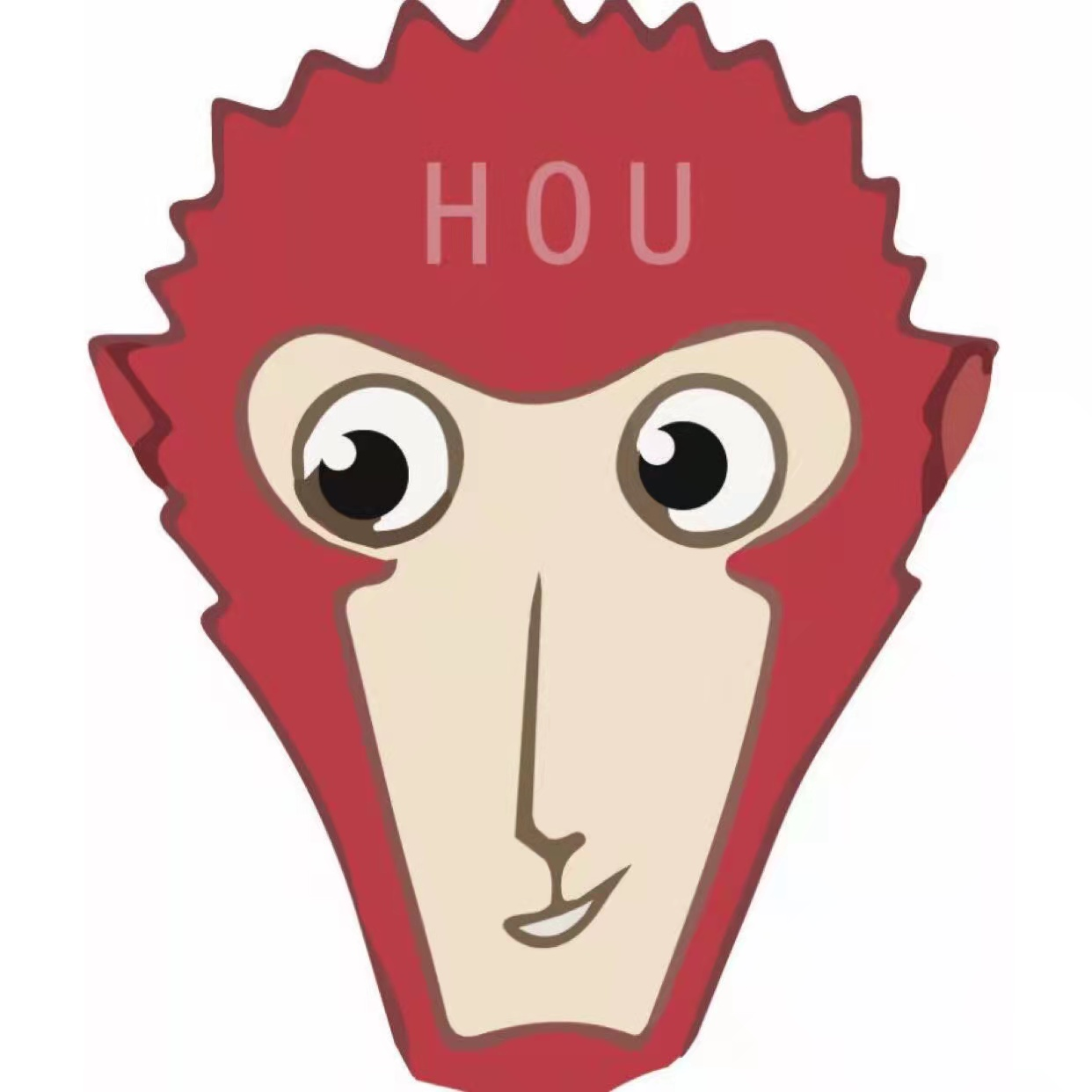某公司的一道机考题的解答

+关注继续查看

昨天看到某个公司招聘出的一道题目，题目是这样的：判断任意三个点是否构成三角形，以及某个点是否位于指定的三角形内。
关于这个问题，我给出了自己的答案，首先解决第一个问题：

/// <summary>

/// IsTriangle 判断集合中的头三个点PointF是否可以构成一个三角形

/// </summary>
public static bool IsTriangle(ArrayList ptList)
{

PointF pt0 = (PointF)ptList ;
PointF pt1
= (PointF)ptList ;
PointF pt2
= (PointF)ptList ;

//如果有两个点相同

if(pt0.Equals(pt1) || pt0.Equals(pt2) || pt1.Equals(pt2) )
{

return false ;
}

float length_01 = (float)Math.Sqrt((pt0.X - pt1.X)*(pt0.X - pt1.X) + (pt0.Y - pt1.Y)*(pt0.Y - pt1.Y)) ;

float length_02 = (float)Math.Sqrt((pt0.X - pt2.X)*(pt0.X - pt2.X) + (pt0.Y - pt2.Y)*(pt0.Y - pt2.Y)) ;

float length_12 = (float)Math.Sqrt((pt2.X - pt1.X)*(pt2.X - pt1.X) + (pt2.Y - pt1.Y)*(pt2.Y - pt1.Y)) ;

bool result0 = (length_01+length_02 <= length_12)  ;

bool result1 = (length_01+length_12 <= length_02)  ;

bool result2 = (length_02+length_12 <= length_01)  ;

if(result0 || result1 || result2)
{

return false ;
}

return true ;
}

该解答分为两步，首先判断是否有重点，接着以两边之和大于第三边作为构成三角形的依据。

关于第二个问题稍微复杂些，不过幸好我在早期研究过并解决了一个更常见的问题，那就是判断一个点是否位于某个多边形内，而且即使这个多边形是凹多边形。这个功能在EnterpriseServerBase.XMath.Geometry.Polygon类中实现。
对于问题二的解答，我封装了Triangle类，它不仅借助Polygon类解决了问题二，而且可以计算三角形的面积和各个边长。public class Triangle{private ArrayList vertextList = null ;private ArrayList lengthList  = null ;private float myArea = 0 ;ctorArea ,GetEdgeLengthContains}

Polygon类的实现比较复杂，代码也比较多，源码就不列出来了，可以点击这里下载。7332 01131 0873 09042 0517 010640 01006 01728 05231 05915 0
zhuweisky

300

0

《Nacos架构&原理》

《看见新力量：二》电子书